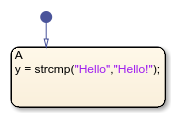# strcmp

Compare strings

## Syntax

``tf = strcmp(s1,s2)``
``s1 == s2``
``s1 != s2``
``tf = strcmp(s1,s2,n)``

## Description

example

````tf = strcmp(s1,s2)` compares strings `s1` and `s2`. Returns `0` if the two strings are identical. Otherwise returns a nonzero integer. The sign of the output value depends on the lexicographic ordering of the input strings `s1` and `s2`.The magnitude of the output value depends on the compiler that you use. This value can differ in simulation and generated code. Strings are considered identical when they have the same size and content.This operator is consistent with the C library function `strcmp` or the C++ function `string.compare`, depending on the compiler that you select for code generation. The operator behaves differently than the function `strcmp` in MATLAB®.```

example

````s1 == s2` is an alternative way to execute `strcmp(s1,s2) == 0`.```

example

````s1 != s2` is an alternative way to execute `strcmp(s1,s2) != 0`.```

example

````tf = strcmp(s1,s2,n)` returns `0` if the first `n` characters in `s1` and `s2` are identical.```

Note

The operator `strcmp` is supported only in Stateflow® charts that use C as the action language.

## Examples

expand all

Return a value of `0` (strings are equal).

`tf = strcmp("abc","abc");`Return a nonzero value (strings are not equal).

`tf = strcmp("abc","abcd");`Return a value of `true`.

`["abc" == "abc"]`Return a value of `true`.

`["abc" != "abcd"]`Return a value of `0` (substrings are equal).

`tf = strcmp("abc","abcd",3);`## Tips

Enclose literal strings with single or double quotes.

### Topics

Introduced in R2018b

## SupportGet trial now